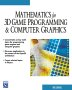# Maths - Progressions

A sequence of 'numbers' or 'quantities' connected in some definite way is called a SERIES and the 'quantity' connected is called a TERM. This page covers:

• Arithmetic Progressions (AP)
• Geometric Progressions (GP)
• Sum to Infinity
• The Binomial Theorem
• Pascals Triangle

## Arithmetic Progressions (AP)

An AP is a series in which each term is formed from the proceeding term by the addition or subtraction of a constant quantity known as the common difference (d).

#### Examples

 2,4,6,8,10 d = 2 16,14,12,10 d = -2 4,0,-4 d = -4

### Equation for the nth term

If we let:

• a = first term
• n = number of terms

the sequence is:

a, a+d, a+2d, a+3d...

So the nth term is:

a+(n-1)d

#### Example

Find the 10th term of 2, 5, 8, 11

10th term = 2+(9*3)

= 29

### To find the sum to n terms of an AP

Let Sn = Sum of 'n' terms, then,

Sn = a + (a+d) + (a+2d) + (a+3d) + ...+ a+(n-1)d

Let l = last term, then,

Sn = a + (a+d) + (a+2d) + (a+3d) + ...+ (l-3d) + (l -2d) + (l - d) + l

reversing the order of this gives:

Sn = l + (l-d) + (l-2d) + (l-3d) + ...+ (a+3d) + (a +2d) + (a + d) + a

Adding these last two equations gives,

2*Sn = a+l + (a+l) + (a+l) + (a+l) + ...+ (a+l) + (a+l) + (a+l) + a+l

2*Sn = (a+l) to n terms

2*Sn = (a+l)*n

Sn = n/2*(a+l)

but l = a+(n-1)d, so substituting this gives:

Sn = n/2*(2a+(n-1)d)

### Arithmetic Mean

When 3 quantities are in arithmetic progression the middle term is the arithmetic mean of the other two.

Find the arithmetic mean of a & b:

Let A be the arithmetic mean, so,

A - a = b - A

2A = a + b

A = (a+b)/2

## Geometric Progressions (GP)

A series of terms, each of which is formed by multiplying the term which proceeds it by a constant factor, known as the common ratio (r)

#### Examples

 3, 6, 12, 24, 48 r = 2 50, 25, 12.5, 6.25 r = 1/2 +9,-27,+81 r = -3

### Equation for the nth term

If we let:

• a = first term
• n = number of terms

the sequence is:

a, ar, ar2, ar3...

So the nth term is:

ar(n-1)

#### Example

Find the 5th term of a GP whose 4th term is 5 and whose 7th term is 320

First find a & r

4th term: ar3 = 5

7th term: ar6 = 320

dividing the 7th term by the 4th term gives:

r3 = 64

therefore:

a = 5/64

r = 3√64 = 4

5th term: ar4 = 5/64 * 44 = 20

### To find the sum to n terms of an GP

Let Sn = Sum of 'n' terms of a series in a GP whose 1stterm is 'a' and common ratio is r.

Sn = a + ar + ar2 + ... + ar(n-2) + ar(n-1)

multiply both sides by r:

r*Sn = ar + ar2 + ar3+ ... + ar(n-1) + arn

subtract the last equation from the one before it:

Sn - r*Sn = a - arn

Sn (1- r)= a (1 - rn)

## Sum to Infinity

It can be shown that:

S= a/(1-r)

#### Example 1

Find the sum to infinity of: 5,-1,1/5...

a = 5

r = -1/5

S= 5/(1-(-1/5)) = 5/(6/5) = 25/6

#### Example 2

The recurring decimal 0.33333 can be written as a series:

0.3 + 0.03 + 0.003 + ...

By finding the sum to infinity express as a fraction:

S= a/(1-r) = 0.3 /(1-1/10) = 0.3/(9/10) = 3/9 = 1/3

## The Binomial Theorem

A binomial expression is a algebraic expression consisting of the sum of the two terms e.g. (a+b), (x-y), (4x2+2y2).

In general any such expression can be denoted by (a+b).

Expansions of (a+b):

(a+b)2= a2+ 2ab + b2

(a+b)3= a3+ 3a2b + 3ab2 + b3

(a+b)4= a4+ 4a3b + 3a2b2+ 4ab3 + b4

It can be seen from the above that the coefficients follow a rule: to find the coefficient for each higher power we add the first and second to get a new second, the second and third to get a new third, the third and fourth to get a new forth and so on.

## Pascals Triangle

(a+b)n= an+ n a(n-1)b + n (n-1)/2! a(n-2) b2+ n (n-1)(n-2)/3! a(n-3)b3 + ... + bn

 n 2n= 0 1 1 1 1 1 1+1 2 1 2 1 1+2+1 3 1 3 3 1 1+3+3+1 4 1 4 6 4 1 1+4+6+4+1 5 1 5 10 10 5 1 1+5+10+10+5+1 6 1 6 15 20 15 6 1 1+6+15+20+15+6+1 7 1 7 21 35 35 21 7 1 1+7+21+35+35+21+7+1 8 1 8 28 56 70 56 28 8 1 1+8+28+56+70+56+28+8+1

when a=1 and b=x the theorem becomes

(1+x)n= 1 + n x + n (n-1)/2! x 2+ n (n-1)(n-2)/3! x 3 + ... + x n

#### example

Expand (2x+3)5

(2x+3)5= (2x)5+ 5(2x)4*3 + (5*4)/(1*2)(2x)3(3)2+(5*4*3)/(1*2*3)(2x)2(3)3+(5*4*3*2)/(1*2*3*4)(2x)(3)4+(5*4*3*2*1)/(1*2*3*4*4)(3)5

 metadata block see also: Correspondence about this page Book Shop - Further reading. Where I can, I have put links to Amazon for books that are relevant to the subject, click on the appropriate country flag to get more details of the book or to buy it from them.Mathematics for 3D game Programming - Includes introduction to Vectors, Matrices, Transforms and Trigonometry. (But no euler angles or quaternions). Also includes ray tracing and some linear & rotational physics also collision detection (but not collision response). Other Math Books Specific to this page here: